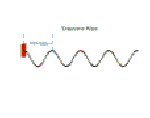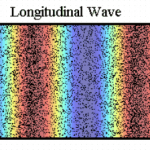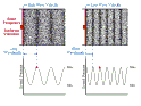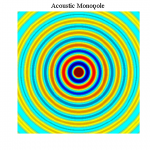## Summary

#### The nature of waves• A wave is a disturbance in a medium.
• It carries energy from one point to another without transferring matter.
• There are two main types: Longitudinal and Transverse.
• Sound waves are an example of longitudinal waves.
• Typically, sound waves travel at about 340 meters per second in air at room temperature and pressure.

#### Ways of showing wavesWe use three ways of representing the propagation of waves:

• Dots.
• Colour maps.
• Line graphs.

#### Wavelength-frequency relationFrequency f, wavelength λ and wave velocity v are mathematically related by:

v = f λ

#### Point sources and inverse-square lawThe energy carried by waves that radiate uniformly in all directions from a point source decay as the inverse of the square of the distance from the point source. This is known as the inverse square law.